# NMR Restraints Grid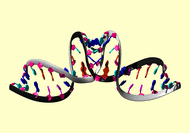Result table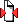(Save to zip file containing files for each block)

 image mrblock_id pdb_id cing stage position program type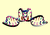476095 1bau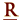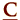cing 1-original 3 AMBER angle

```######################################
# RNA-DIMER Hydrogen bond restraints #
######################################

# G1-C23
# flat angle constraint number   1
&rst  iat =  1, 23, 23, iresid = 1,
atnam(1)='O6   ',atnam(2)='H41 ',atnam(3)='N4   ',
r1 = 160.0, r2 = 170.0, r3 = 190.0, r4 = 210.0,
rk2 =30.001, rk3 =30.001, &end
# flat angle constraint number   2
&rst  iat =  1,  1, 23, iresid = 1,
atnam(1)='N1   ',atnam(2)='H1   ',atnam(3)='N3   ',
r1 = 160.0, r2 = 170.0, r3 = 190.0, r4 = 210.0,
rk2 =30.001, rk3 =30.001, &end
# flat angle constraint number   3
&rst  iat =  1,  1, 23, iresid = 1,
atnam(1)='N2   ',atnam(2)='H21 ',atnam(3)='O2   ',
r1 = 160.0, r2 = 170.0, r3 = 190.0, r4 = 210.0,
rk2 =30.001, rk3 =30.001, &end
# G2-C22
# flat angle constraint number   1
&rst  iat =  2, 22, 22, iresid = 1,
atnam(1)='O6   ',atnam(2)='H41 ',atnam(3)='N4   ',
r1 = 160.0, r2 = 170.0, r3 = 190.0, r4 = 210.0,
rk2 =30.001, rk3 =30.001, &end
# flat angle constraint number   2
&rst  iat =  2,  2, 22, iresid = 1,
atnam(1)='N1   ',atnam(2)='H1   ',atnam(3)='N3   ',
r1 = 160.0, r2 = 170.0, r3 = 190.0, r4 = 210.0,
rk2 =30.001, rk3 =30.001, &end
# flat angle constraint number   3
&rst  iat =  2,  2, 22, iresid = 1,
atnam(1)='N2   ',atnam(2)='H21 ',atnam(3)='O2   ',
r1 = 160.0, r2 = 170.0, r3 = 190.0, r4 = 210.0,
rk2 =30.001, rk3 =30.001, &end

# C3-G21
# flat angle constraint number   4
&rst  iat =  21,  3,  3, iresid = 1,
atnam(1)='O6   ',atnam(2)='H41 ',atnam(3)='N4   ',
r1 = 160.0, r2 = 170.0, r3 = 190.0, r4 = 210.0,
rk2 =30.001, rk3 =30.001, &end
# flat angle constraint number   5
&rst  iat = 21, 21,  3, iresid = 1,
atnam(1)='N1   ',atnam(2)='H1   ',atnam(3)='N3   ',
r1 = 160.0, r2 = 170.0, r3 = 190.0, r4 = 210.0,
rk2 =30.001, rk3 =30.001, &end
# flat angle constraint number   6
&rst  iat = 21, 21,  3, iresid = 1,
atnam(1)='N2   ',atnam(2)='H21 ',atnam(3)='O2   ',
r1 = 160.0, r2 = 170.0, r3 = 190.0, r4 = 210.0,
rk2 =30.001, rk3 =30.001, &end

# A4-U20
# flat angle constraint number   10
&rst  iat =  4, 20, 20, iresid = 1,
atnam(1)='N1   ',atnam(2)='H3   ',atnam(3)='N3   ',
r1 = 160.0, r2 = 170.0, r3 = 190.0, r4 = 210.0,
rk2 =30.001, rk3 =30.001, &end
# flat angle constraint number   11
&rst  iat =  4,  4, 20, iresid = 1,
atnam(1)='N6   ',atnam(2)='H61 ',atnam(3)='O4   ',
r1 = 160.0, r2 = 170.0, r3 = 190.0, r4 = 210.0,
rk2 =30.001, rk3 =30.001, &end

# A5-U19
# flat angle constraint number   10
&rst  iat =  5, 19, 19, iresid = 1,
atnam(1)='N1   ',atnam(2)='H3   ',atnam(3)='N3   ',
r1 = 160.0, r2 = 170.0, r3 = 190.0, r4 = 210.0,
rk2 =30.001, rk3 =30.001, &end
# flat angle constraint number   11
&rst  iat =  5,  5, 19, iresid = 1,
atnam(1)='N6   ',atnam(2)='H61 ',atnam(3)='O4   ',
r1 = 160.0, r2 = 170.0, r3 = 190.0, r4 = 210.0,
rk2 =30.001, rk3 =30.001, &end

############################################
# SECOND HAIR-PIN Hydrogen bond restraints #
############################################

# G24-C46
# flat angle constraint number   1
&rst  iat =  24, 46, 46, iresid = 1,
atnam(1)='O6   ',atnam(2)='H41 ',atnam(3)='N4   ',
r1 = 160.0, r2 = 170.0, r3 = 190.0, r4 = 210.0,
rk2 =30.001, rk3 =30.001, &end
# flat angle constraint number   2
&rst  iat =  24, 24, 46, iresid = 1,
atnam(1)='N1   ',atnam(2)='H1   ',atnam(3)='N3   ',
r1 = 160.0, r2 = 170.0, r3 = 190.0, r4 = 210.0,
rk2 =30.001, rk3 =30.001, &end
# flat angle constraint number   3
&rst  iat =  24, 24, 46, iresid = 1,
atnam(1)='N2   ',atnam(2)='H21 ',atnam(3)='O2   ',
r1 = 160.0, r2 = 170.0, r3 = 190.0, r4 = 210.0,
rk2 =30.001, rk3 =30.001, &end

# G25-C45
# flat angle constraint number   1
&rst  iat =  25, 45, 45, iresid = 1,
atnam(1)='O6   ',atnam(2)='H41 ',atnam(3)='N4   ',
r1 = 160.0, r2 = 170.0, r3 = 190.0, r4 = 210.0,
rk2 =30.001, rk3 =30.001, &end
# flat angle constraint number   2
&rst  iat =  25, 25, 45, iresid = 1,
atnam(1)='N1   ',atnam(2)='H1   ',atnam(3)='N3   ',
r1 = 160.0, r2 = 170.0, r3 = 190.0, r4 = 210.0,
rk2 =30.001, rk3 =30.001, &end
# flat angle constraint number   3
&rst  iat =  25, 25, 45, iresid = 1,
atnam(1)='N2   ',atnam(2)='H21 ',atnam(3)='O2   ',
r1 = 160.0, r2 = 170.0, r3 = 190.0, r4 = 210.0,
rk2 =30.001, rk3 =30.001, &end

# C26-G44
# flat angle constraint number   4
&rst  iat =  44, 26, 26, iresid = 1,
atnam(1)='O6   ',atnam(2)='H41 ',atnam(3)='N4   ',
r1 = 160.0, r2 = 170.0, r3 = 190.0, r4 = 210.0,
rk2 =30.001, rk3 =30.001, &end
# flat angle constraint number   5
&rst  iat = 44, 44, 26, iresid = 1,
atnam(1)='N1   ',atnam(2)='H1   ',atnam(3)='N3   ',
r1 = 160.0, r2 = 170.0, r3 = 190.0, r4 = 210.0,
rk2 =30.001, rk3 =30.001, &end
# flat angle constraint number   6
&rst  iat = 44, 44, 26, iresid = 1,
atnam(1)='N2   ',atnam(2)='H21 ',atnam(3)='O2   ',
r1 = 160.0, r2 = 170.0, r3 = 190.0, r4 = 210.0,
rk2 =30.001, rk3 =30.001, &end

# A27-U43
# flat angle constraint number   10
&rst  iat =  27, 43, 43, iresid = 1,
atnam(1)='N1   ',atnam(2)='H3   ',atnam(3)='N3   ',
r1 = 160.0, r2 = 170.0, r3 = 190.0, r4 = 210.0,
rk2 =30.001, rk3 =30.001, &end
# flat angle constraint number   11
&rst  iat =  27,  27, 43, iresid = 1,
atnam(1)='N6   ',atnam(2)='H61 ',atnam(3)='O4   ',
r1 = 160.0, r2 = 170.0, r3 = 190.0, r4 = 210.0,
rk2 =30.001, rk3 =30.001, &end

# A28-U42
# flat angle constraint number   10
&rst  iat =  28, 42, 42, iresid = 1,
atnam(1)='N1   ',atnam(2)='H3   ',atnam(3)='N3   ',
r1 = 160.0, r2 = 170.0, r3 = 190.0, r4 = 210.0,
rk2 =30.001, rk3 =30.001, &end
# flat angle constraint number   11
&rst  iat =  28,  28, 42, iresid = 1,
atnam(1)='N6   ',atnam(2)='H61 ',atnam(3)='O4   ',
r1 = 160.0, r2 = 170.0, r3 = 190.0, r4 = 210.0,
rk2 =30.001, rk3 =30.001, &end

###############################
# LOOP DUPLEX FLAT ANGLE RESTRAINTS #
###############################

# G10-C38
# flat angle constraint number   12
&rst  iat =  10, 38, 38, iresid = 1,
atnam(1)='O6   ',atnam(2)='H41 ',atnam(3)='N4   ',
r1 = 160.0, r2 = 170.0, r3 = 190.0, r4 = 210.0,
rk2 =30.001, rk3 =30.001, &end
# flat angle constraint number   13
&rst  iat =  10,  10, 38, iresid = 1,
atnam(1)='N1   ',atnam(2)='H1   ',atnam(3)='N3   ',
r1 = 160.0, r2 = 170.0, r3 = 190.0, r4 = 210.0,
rk2 =30.001, rk3 =30.001, &end
# flat angle constraint number   14
&rst  iat =  10,  10, 38, iresid = 1,
atnam(1)='N2   ',atnam(2)='H21 ',atnam(3)='O2   ',
r1 = 160.0, r2 = 170.0, r3 = 190.0, r4 = 210.0,
rk2 =30.001, rk3 =30.001, &end
# C11-G37
# flat angle constraint number   4
&rst  iat =  37, 11, 11, iresid = 1,
atnam(1)='O6   ',atnam(2)='H41 ',atnam(3)='N4   ',
r1 = 160.0, r2 = 170.0, r3 = 190.0, r4 = 210.0,
rk2 =30.001, rk3 =30.001, &end
# flat angle constraint number   5
&rst  iat = 37, 37, 11, iresid = 1,
atnam(1)='N1   ',atnam(2)='H1   ',atnam(3)='N3   ',
r1 = 160.0, r2 = 170.0, r3 = 190.0, r4 = 210.0,
rk2 =30.001, rk3 =30.001, &end
# flat angle constraint number   6
&rst  iat = 37, 37, 11, iresid = 1,
atnam(1)='N2   ',atnam(2)='H21 ',atnam(3)='O2   ',
r1 = 160.0, r2 = 170.0, r3 = 190.0, r4 = 210.0,
rk2 =30.001, rk3 =30.001, &end

# G12-C36
# flat angle constraint number   12
&rst  iat =  12, 36, 36, iresid = 1,
atnam(1)='O6   ',atnam(2)='H41 ',atnam(3)='N4   ',
r1 = 160.0, r2 = 170.0, r3 = 190.0, r4 = 210.0,
rk2 =30.001, rk3 =30.001, &end
# flat angle constraint number   13
&rst  iat =  12,  12, 36, iresid = 1,
atnam(1)='N1   ',atnam(2)='H1   ',atnam(3)='N3   ',
r1 = 160.0, r2 = 170.0, r3 = 190.0, r4 = 210.0,
rk2 =30.001, rk3 =30.001, &end
# flat angle constraint number   14
&rst  iat =  12,  12, 36, iresid = 1,
atnam(1)='N2   ',atnam(2)='H21 ',atnam(3)='O2   ',
r1 = 160.0, r2 = 170.0, r3 = 190.0, r4 = 210.0,
rk2 =30.001, rk3 =30.001, &end

# C13-G35
# flat angle constraint number   4
&rst  iat =  35, 13, 13, iresid = 1,
atnam(1)='O6   ',atnam(2)='H41 ',atnam(3)='N4   ',
r1 = 160.0, r2 = 170.0, r3 = 190.0, r4 = 350.0,
rk2 =30.001, rk3 =30.001, &end
# flat angle constraint number   5
&rst  iat = 35, 35, 13, iresid = 1,
atnam(1)='N1   ',atnam(2)='H1   ',atnam(3)='N3   ',
r1 = 160.0, r2 = 170.0, r3 = 190.0, r4 = 350.0,
rk2 =30.001, rk3 =30.001, &end
# flat angle constraint number   6
&rst  iat = 35, 35, 13, iresid = 1,
atnam(1)='N2   ',atnam(2)='H21 ',atnam(3)='O2   ',
r1 = 160.0, r2 = 170.0, r3 = 190.0, r4 = 350.0,
rk2 =30.001, rk3 =30.001, &end

# G14-C34
# flat angle constraint number   12
&rst  iat =  14, 34, 34, iresid = 1,
atnam(1)='O6   ',atnam(2)='H41 ',atnam(3)='N4   ',
r1 = 160.0, r2 = 170.0, r3 = 190.0, r4 = 210.0,
rk2 =30.001, rk3 =30.001, &end
# flat angle constraint number   13
&rst  iat =  14,  14, 34, iresid = 1,
atnam(1)='N1   ',atnam(2)='H1   ',atnam(3)='N3   ',
r1 = 160.0, r2 = 170.0, r3 = 190.0, r4 = 210.0,
rk2 =30.001, rk3 =30.001, &end
# flat angle constraint number   14
&rst  iat =  14,  14, 34, iresid = 1,
atnam(1)='N2   ',atnam(2)='H21 ',atnam(3)='O2   ',
r1 = 160.0, r2 = 170.0, r3 = 190.0, r4 = 210.0,
rk2 =30.001, rk3 =30.001, &end

# C15-G21
# flat angle constraint number   4
&rst  iat =  33, 15, 15, iresid = 1,
atnam(1)='O6   ',atnam(2)='H41 ',atnam(3)='N4   ',
r1 = 160.0, r2 = 170.0, r3 = 190.0, r4 = 210.0,
rk2 =30.001, rk3 =30.001, &end
# flat angle constraint number   5
&rst  iat = 33, 33, 15, iresid = 1,
atnam(1)='N1   ',atnam(2)='H1   ',atnam(3)='N3   ',
r1 = 160.0, r2 = 170.0, r3 = 190.0, r4 = 210.0,
rk2 =30.001, rk3 =30.001, &end
# flat angle constraint number   6
&rst  iat = 33, 33, 15, iresid = 1,
atnam(1)='N2   ',atnam(2)='H21 ',atnam(3)='O2   ',
r1 = 160.0, r2 = 170.0, r3 = 190.0, r4 = 210.0,
rk2 =30.001, rk3 =30.001, &end

```

Please acknowledge these references in publications where the data from this site have been utilized.

Contact the webmaster for help, if required. Wednesday, October 20, 2021 11:17:06 PM GMT (wattos1)